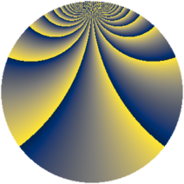# Properties

 Label 1805.2.bfLevel $1805$ Weight $2$ Character orbit 1805.bf Rep. character $\chi_{1805}(4,\cdot)$ Character field $\Q(\zeta_{342})$ Dimension $20304$ Sturm bound $380$

# Related objects

## Defining parameters

 Level: $$N$$ $$=$$ $$1805 = 5 \cdot 19^{2}$$ Weight: $$k$$ $$=$$ $$2$$ Character orbit: $$[\chi]$$ $$=$$ 1805.bf (of order $$342$$ and degree $$108$$) Character conductor: $$\operatorname{cond}(\chi)$$ $$=$$ $$1805$$ Character field: $$\Q(\zeta_{342})$$ Sturm bound: $$380$$

## Dimensions

The following table gives the dimensions of various subspaces of $$M_{2}(1805, [\chi])$$.

Total New Old
Modular forms 20736 20736 0
Cusp forms 20304 20304 0
Eisenstein series 432 432 0

## Trace form

 $$20304 q - 210 q^{4} - 108 q^{5} - 222 q^{6} - 216 q^{9} + O(q^{10})$$ $$20304 q - 210 q^{4} - 108 q^{5} - 222 q^{6} - 216 q^{9} - 99 q^{10} - 222 q^{11} - 234 q^{14} - 117 q^{15} - 186 q^{16} - 240 q^{19} - 132 q^{20} - 174 q^{21} - 252 q^{24} - 126 q^{25} - 216 q^{26} - 228 q^{29} - 132 q^{30} - 186 q^{31} - 192 q^{34} - 120 q^{35} - 246 q^{36} - 180 q^{39} - 180 q^{40} - 234 q^{41} - 222 q^{44} - 120 q^{45} - 222 q^{46} - 750 q^{49} - 96 q^{50} - 336 q^{51} - 252 q^{54} - 114 q^{55} - 264 q^{56} - 192 q^{59} - 276 q^{61} - 768 q^{64} - 96 q^{65} - 408 q^{66} + 732 q^{69} - 447 q^{70} - 204 q^{71} - 426 q^{74} - 186 q^{75} - 294 q^{76} - 276 q^{79} - 75 q^{80} - 150 q^{81} - 174 q^{84} - 30 q^{85} + 840 q^{86} - 216 q^{89} - 132 q^{90} - 84 q^{91} - 156 q^{94} + 177 q^{95} - 132 q^{96} - 192 q^{99} + O(q^{100})$$

## Decomposition of $$S_{2}^{\mathrm{new}}(1805, [\chi])$$ into newform subspaces

The newforms in this space have not yet been added to the LMFDB.